# Geometric Properties of Objects Chapter Exam

Exam Instructions:

Choose your answers to the questions and click 'Next' to see the next set of questions. You can skip questions if you would like and come back to them later with the yellow "Go To First Skipped Question" button. When you have completed the practice exam, a green submit button will appear. Click it to see your results. Good luck!

### Page 1

#### Question 1 1. Josh claims that these triangles are similar by SSS. Do you agree or disagree with Josh?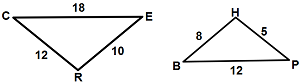#### Question 2 2. Triangle HYV and triangle AYB are similar by the AA similarity theorem. What is the value of x?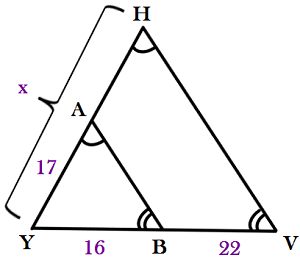#### Question 3 3. In the figure below, Angle 13 = 10y and Angle 11 = 3y + 11. Determine the measure of Angle 16.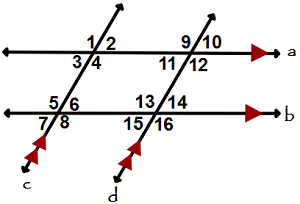#### Question 5 5. Which two lines are parallel?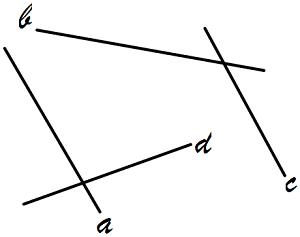### Page 2

#### Question 7 7. Consider the figure below, where Angle 4 = 12m - 4 Angle 5 = 10m + 20 Calculate the measure of Angle 5.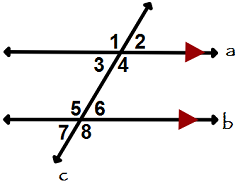#### Question 8 8. What is the scale factor from the smaller rectangle to the larger rectangle pictured below?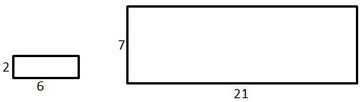#### Question 9 9. What is the area of this trapezoid?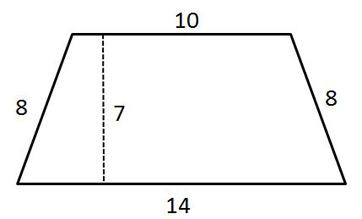### Page 3

#### Question 11 11. Study the given diagram. Find the value of x, the distance between T and E.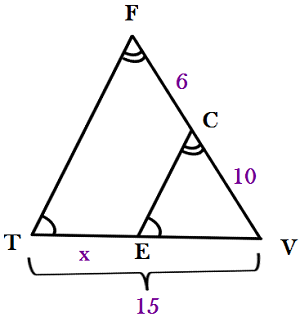#### Question 12 12. What is the relationship between Angle 4 and Angle 1?#### Question 15 15. Given the figure below, Angle 3 = 140y and Angle 5 = 80y + 70. Determine the value of y.### Page 4

#### Question 16 16. The pictured shape is a: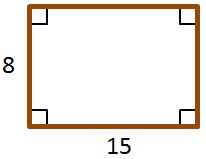#### Question 18 18. What is the perimeter of the pictured square?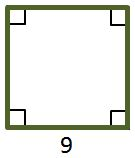#### Question 20 20. If the two triangles pictured below are congruent, then which angles are the same?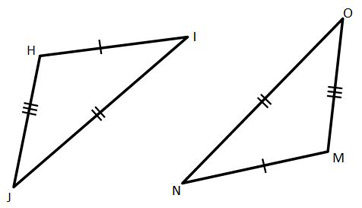### Page 5

#### Question 22 22. Conclude whether triangle BEN is similar to triangle MAT.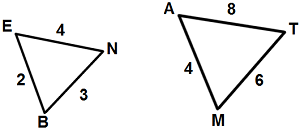#### Question 23 23. If triangle MNO is congruent to triangle PQR, then what is the measure of angle x?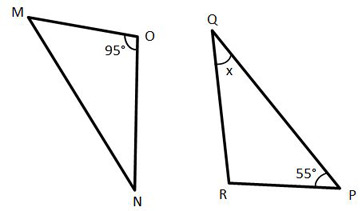#### Question 24 24. If we apply a scale factor of 4 to the triangular prism below, what will the volume of the new prism be?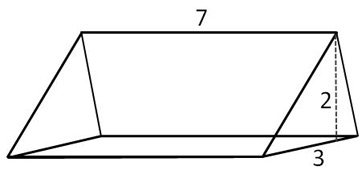### Page 6

#### Question 26 26. In the similar rectangles pictured below, what is y?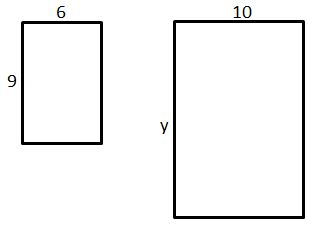#### Question 28 28. Which answer choice is similar to the triangle pictured below?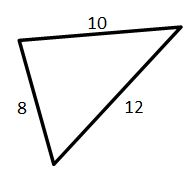#### Question 29 29. Is triangle ABC similar to triangle DEF?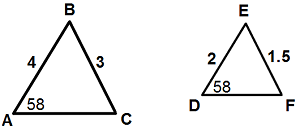#### Question 30 30. The pictured shape is a: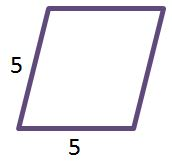#### Geometric Properties of Objects Chapter Exam Instructions

Choose your answers to the questions and click 'Next' to see the next set of questions. You can skip questions if you would like and come back to them later with the yellow "Go To First Skipped Question" button. When you have completed the practice exam, a green submit button will appear. Click it to see your results. Good luck!

Support